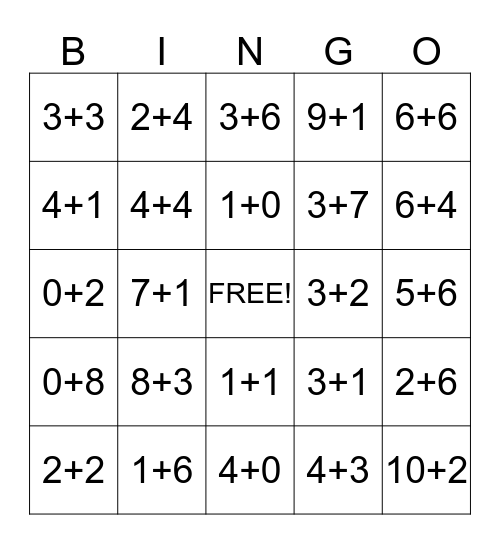This bingo card has a free space and 24 words: 8+3, 1+6, 1+0, 2+2, 6+6, 3+3, 4+1, 2+4, 3+2, 1+1, 3+7, 0+2, 3+1, 2+6, 4+4, 4+3, 5+6, 3+6, 6+4, 7+1, 10+2, 0+8, 9+1 and 4+0.

## Play Online